# Class 7 Maths NCERT Solutions for Chapter – 4 Simple Equations EX – 4.3

## Simple Equations

Question 1.
Solve the following equations :Solution: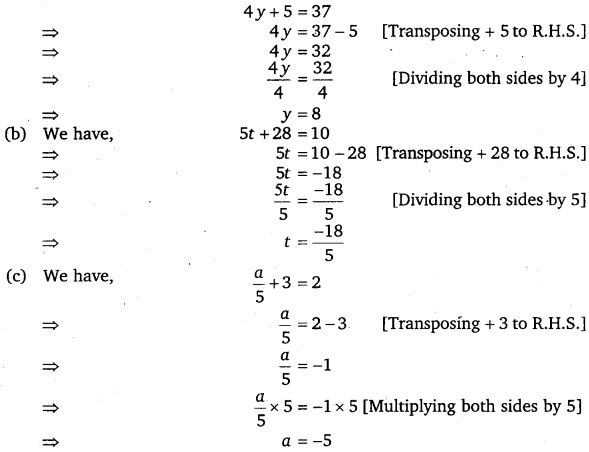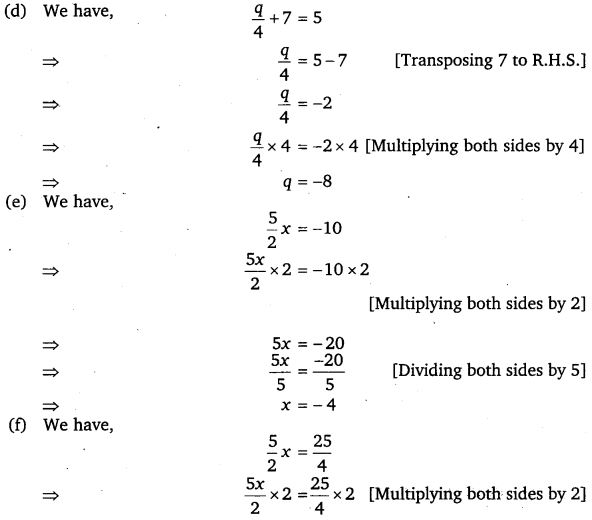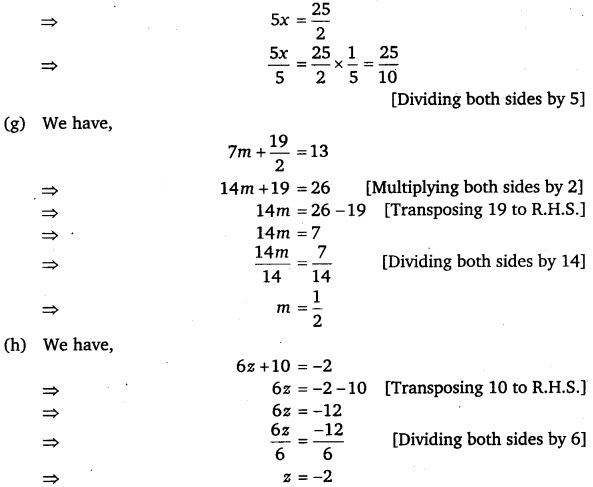Question 2.
Solve the following equations :
(a)
2 (x + 4) = 12
(b) 3 (n – 5) = 21
(c) 3 (n – 5) = -21
(d) -4 (2 + x) = 8
(e) 4 (2 – x) = 8

Solution:

Question 3.
Solve the following equations :
(a)
4 = 5 (p – 2)
(b) -4 = 5 (p – 2)
(c) 16 = 4 + 3 (t + 2)
(d) 4 + 5 (p – 1) = 34
(e) 0 = 16 + 4 (m – 6)

Solution: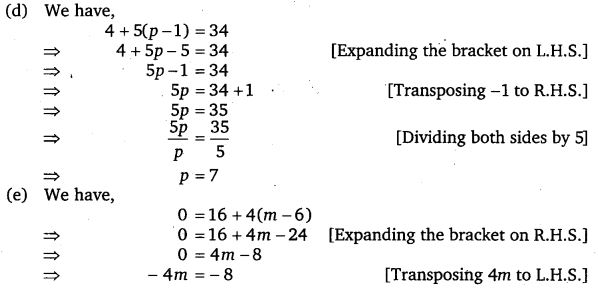Question 4.
(a) Construct 3 equations starting with x = 2.
(b) Construct 3 equations starting with x = -2.

Solution:
(a) First equation :
Multiply both sides by 3, 3x = 6
Add 2 to both sides, 3x + 2 = 8

Second equation :
Multiply both sides by -3, -3x, = -6
Add 8 to both sides, 8 – 3x = 2.

Third equation :
Divide both sides by 5,$\frac { x }{ 5 }$ =$\frac { 2 }{ 5 }$
Subtract 2 from both sides,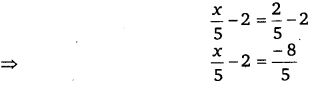(b) First equation :
Multiply both sides by 2, 2x = -4
Subtract 3 from both sides, 2x -3 = -7

Second equation :
Multiply both sides by – 5, – 5x = 10
Add 10 to both sides, 10 – 5x = 20

Third equation :
Divide both sides by 2,$\frac { x }{ 2 }$ = -1
Add 3 to both sides,$\frac { x }{ 2 }$ + 3 = 2